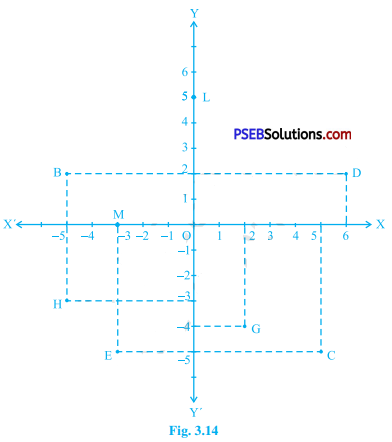# PSEB 9th Class Maths Solutions Chapter 3 Coordinate Geometry Ex 3.2

Punjab State Board PSEB 9th Class Maths Book Solutions Chapter 3 Coordinate Geometry Ex 3.2 Textbook Exercise Questions and Answers.

## PSEB Solutions for Class 9 Maths Chapter 3 Coordinate Geometry Ex 3.2

Question 1.
Write the answer of each of the following questions:
(i) What is the name of horizontal and the vertical lines drawn to determine the position of any point in the Cartesian plane?
The horizontal line and the vertical line drawn in the Cartesian plane to determine the position of any point are named as the x-axis and the y-axis respectively.

(ii) What is the name of each part of the plane formed by these two lines?
These two lines (x-axis and y-axis) partition the Cartesian plane into four parts each of which is called a quadrant. They are named as Quadrant 1, Quadrant 2, Quadrant 3 and Quadrant 4.(iii) Write the name of the point where these two lines intersect.
These two lines (x-axis and y-axis) intersect at the point named as the Origin.

Question 2.
See the figure given below and write the following:(i) The coordinates of B.
(- 5, 2)

(ii) The coordinates of C.
(5, – 5)(iii) The point identified by the coordinates (-3, -5).
E

(iv) The point identified by the coordinates (2, -4).
G

(v) The abscissa of the point D.
6

(vi) The ordinate of the point H.